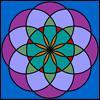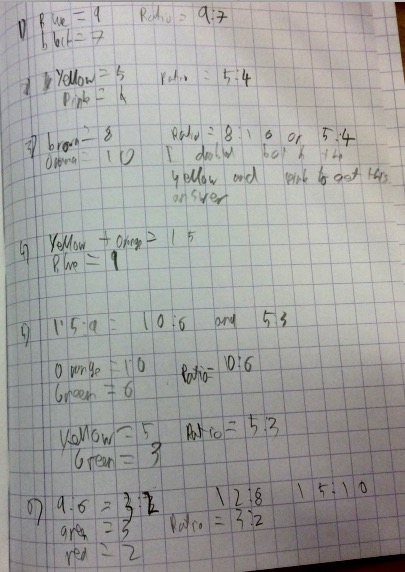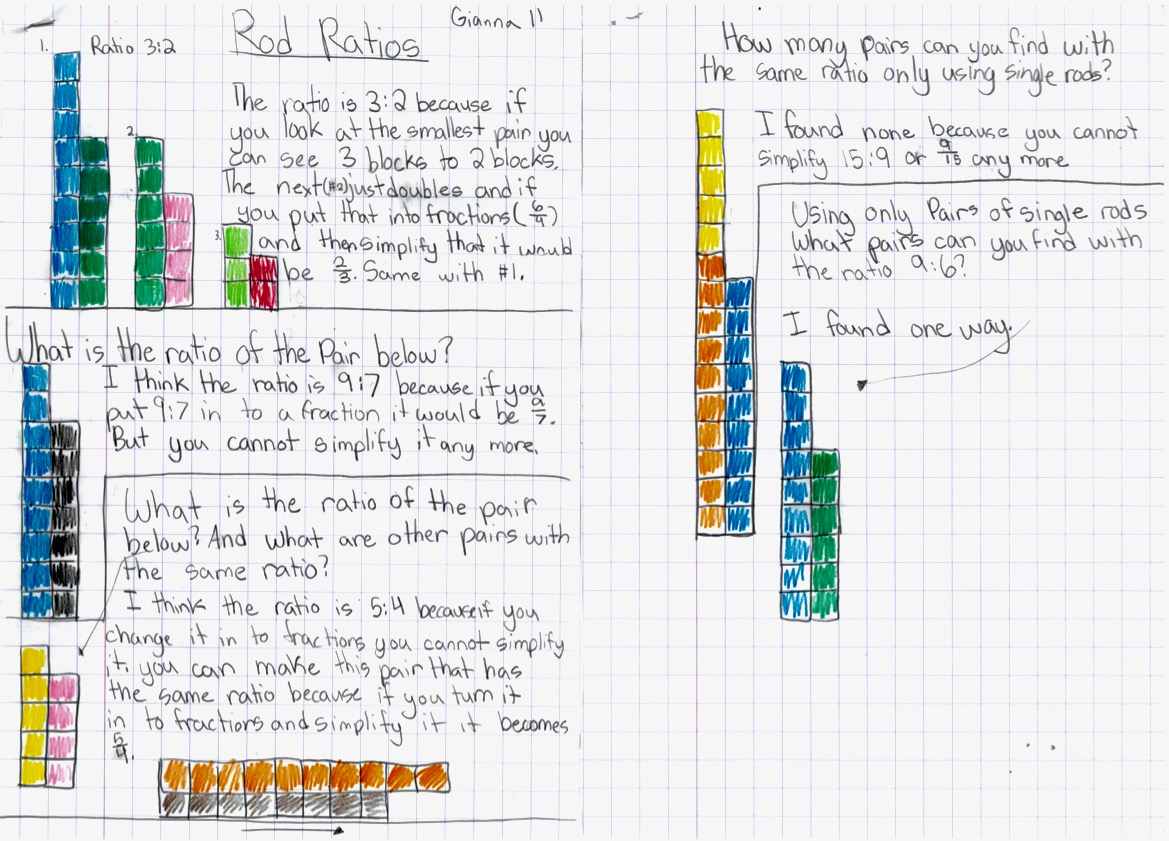#### You may also like### Teddy Town

There are nine teddies in Teddy Town - three red, three blue and three yellow. There are also nine houses, three of each colour. Can you put them on the map of Teddy Town according to the rules?### Overlapping Circles

What shaped overlaps can you make with two circles which are the same size?### Four Triangles Puzzle

Cut four triangles from a square as shown in the picture. How many different shapes can you make by fitting the four triangles back together?

# Rod Ratios

##### Age 7 to 11 Challenge Level:

Monty from the British School in Brussels in Belgium said:

I decided to use the grid mode and count the squares in order to work out the ratios. Here are some of my answers:Gianna, also from the International School of Brussels in Belgium sent in this good piece of work:Well done also to Isis (South Island School), Rohaan (Long Bay Primary) and Samantha (The Hamlin School) for completing this task! Samantha explained how she manged to find a pair with the same ratio as the yellow and pink rods:

The pink rod had 4 units and the yellow one had 5 units. This meant that they had a ratio of 4:5. I then needed to find a like ratio. This can be done by multiplying both numbers in the ratio by the same number. In this case the number had to be 2 because the longest rod is 10 units. So the 8 (brown coloured) and the 10 (orange coloured) rods would have the same ratio as the 4 (pink coloured) and the 5 (yellow coloured).

She also found pairs with the same ratio as the combined yellow+orange to blue:

The ratio of yellow (5) + orange (10) to blue (9) is equal to the ratio 15 to 9. This ratio can be simplified to a 5:3 ratio by dividing both 15 and 9 by 3. Therefore, the yellow and green rods form an equal ratio to that of the yellow + orange to the blue. Another equal ratio would be 10 to 6. Therefore, the dark green and orange rods also form an equal ratio.

Finally she noted that the rods with the same ratio as 9:6 have already been given!
The ratio of 9:6 can be simplified to the ratio 3:2. The possible combinations with rods for that ratios are shown at the start of the question!

Thank you to all those who sent in their work, thoughts and ideas.